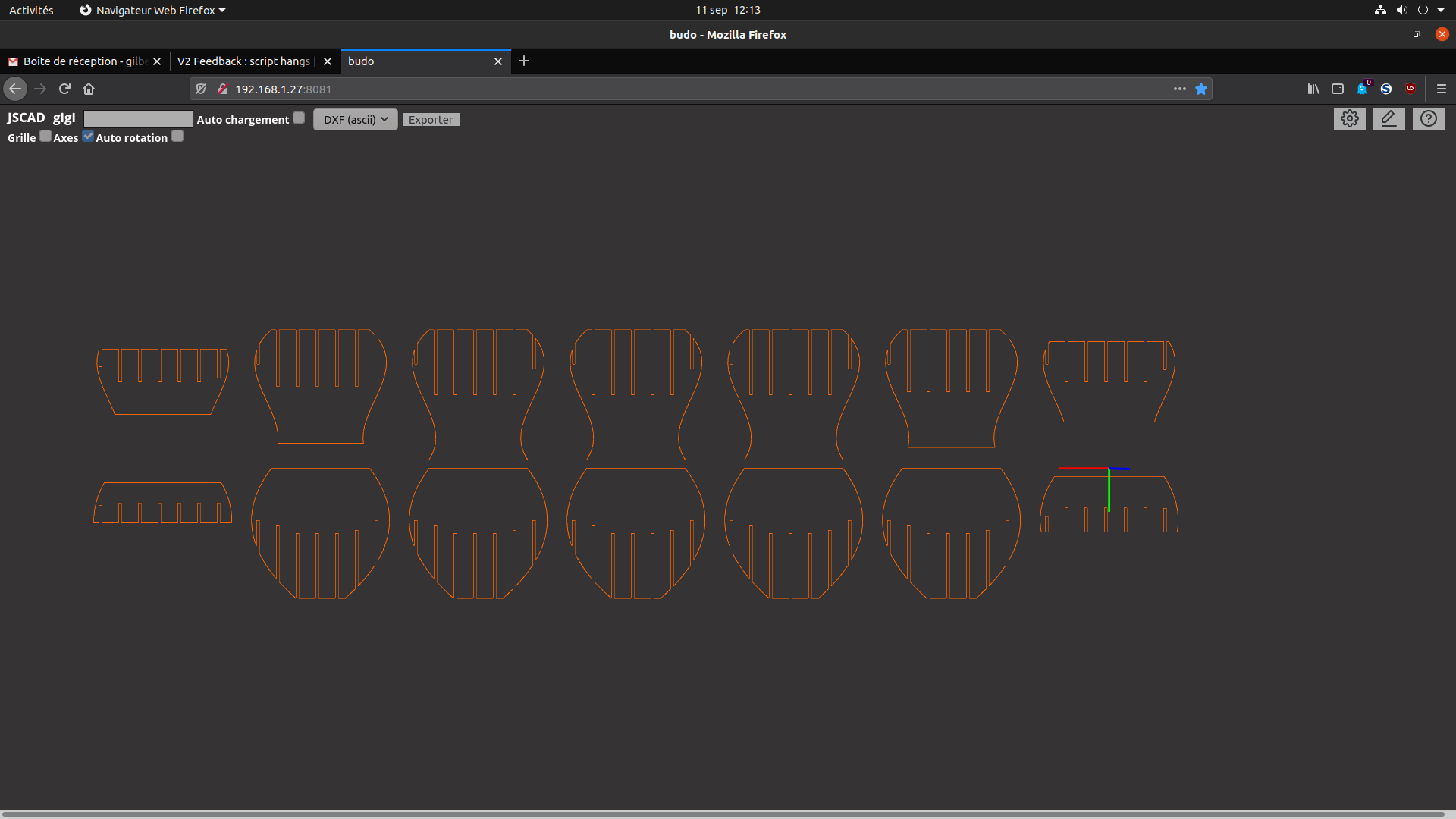# V2 Feedback : script hangs

• Hello, I just updated to last V2 version (alpha 6) and my converted script that worked well with previous update now hangs (keep running after 10 minutes).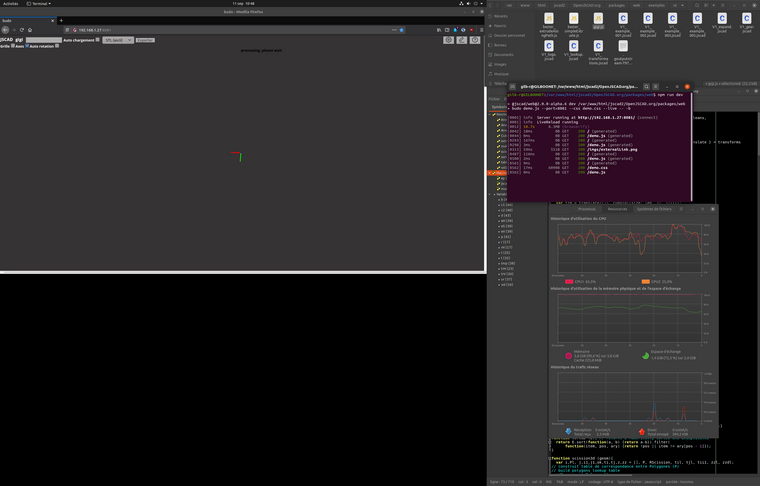The script is here : https://github.com/gilboonet/gilboonet.github.io/blob/master/demos/gigi.js

• @z3dev You're right, the problem was about winding and by correcting it for MeasureArea() < 0 and triangulating was enough to make it work. I updated my source here : https://github.com/gilboonet/gilboonet.github.io/blob/master/demos/gigi.js It contains a volume exported from Wings3d, with Cube1_default() that contains data with quads and table() with this :

``````function table() {

var a = Cube1_default();

var tmp = [];
for(var i = 0; i < a.polygons.length; i++){
if (measureArea(a.polygons[i]) < 0){
a.polygons[i] = a.polygons[i].reverse();
}
for(var j = 1; j< a.polygons[i].length-1; j++){
tmp.push([a.polygons[i], a.polygons[i][j], a.polygons[i][j+1]]);
}
}

return scale([8,8,8], polyhedron({points:a.points, faces:tmp}));

}

``````

There is certainly a way to write this code a more modern way with map() to speed it up.

• @gilboonet can you provide an example of the quad polygons? we may be able to fix this when converting from OBJ files.

• @gilboonet very nice!

it seems that the issues were about the winding of the 2D geometries, and correcting geometries to wind CCW about the Z axis. is that correct? Any bugs that we need to look at?

• I did the version with the little code that modify a polyhedron polygons if needed (first it checks the winding using measureArea() as I just read on a recent issue on github, then it turns it to triangles) . And it's nice to be able to have 3d and 2d rendered together.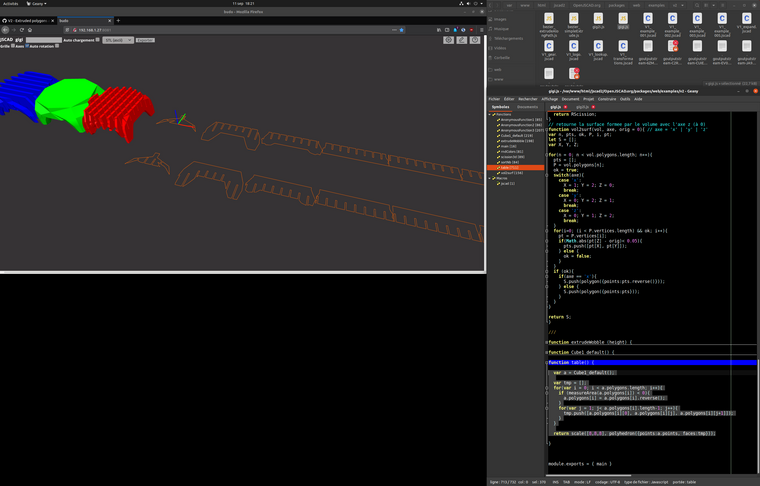• I have it, the polyhedron that I exported from Wings3d to .jscad had quads, hopefully there's an option to export tris and it works with such polyhedron. About polyhedra with polygons instead of triangles, I now use a little code to do the conversion when reading a .obj file on my vanilla js unfolder, but didn't try it with jscad. I will try it with this example.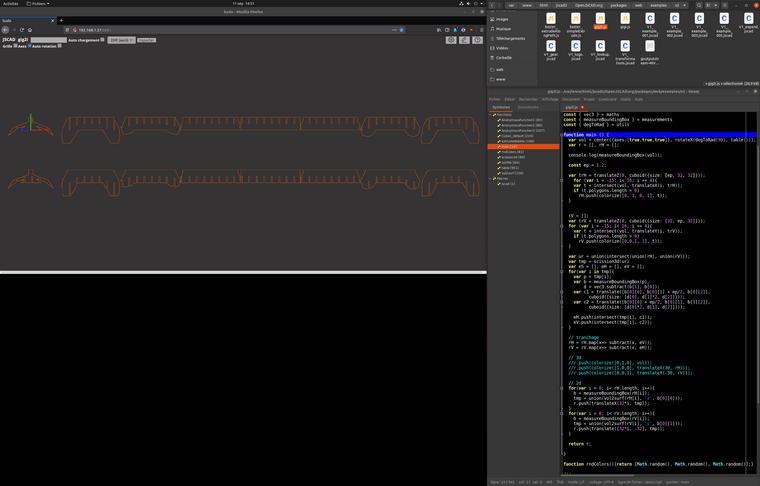• It seems to be an intersect problem, I try to get the intersection between my volume ( a polyhedron) and a cuboid and it hangs. Are there rules for CSG boolean operations ?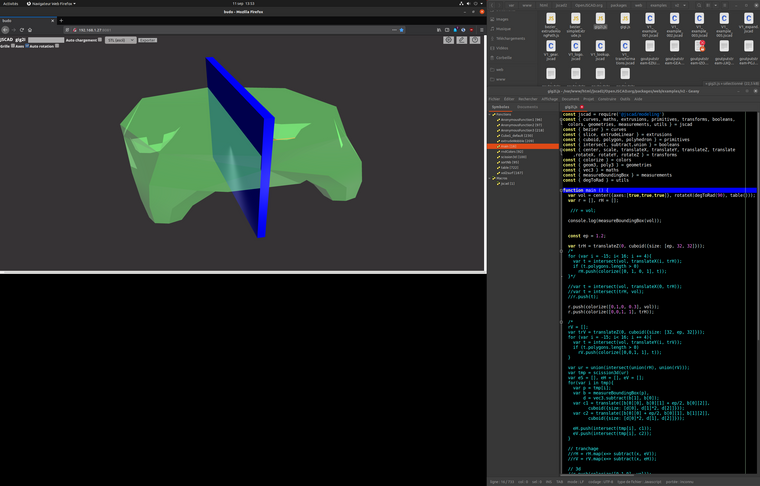• With my first example which uses the extrudeWobble() from BezierSimpleExtrude.jscad example, it works, it is with a polyhedron that is more complex that it hangs, I will try to reduce it.
(working example rendered with alpha 6)